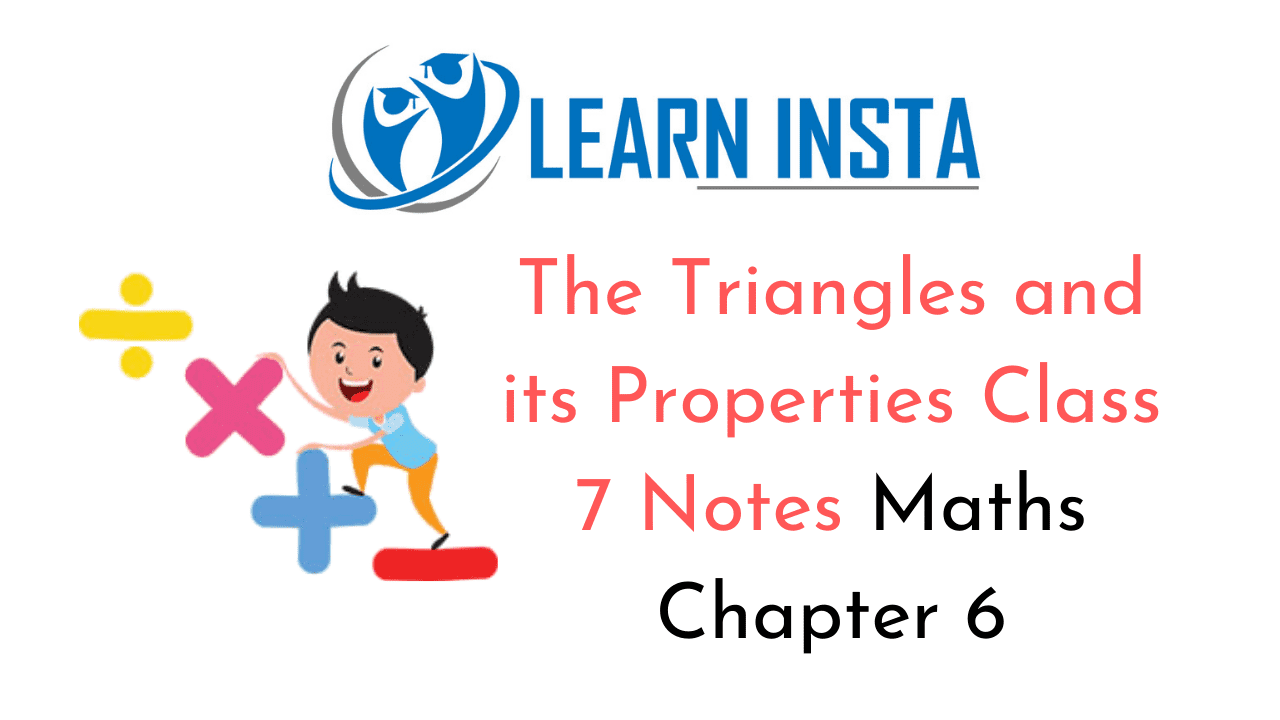On this page, you will find The Triangles and its Properties Class 7 Notes Maths Chapter 6 Pdf free download. CBSE NCERT Class 7 Maths Notes Chapter 6 The Triangles and its Properties will seemingly help them to revise the important concepts in less time.

## CBSE Class 7 Maths Chapter 6 Notes The Triangles and its Properties

### The Triangles and its Properties Class 7 Notes Conceptual Facts

1. A triangle is a simple closed figure made up of three line segments.

2. ΔABC has three sides AB, BC and CA and three angles ∠ABC, ∠BCA and ∠CAB. These are called six elements of the triangle.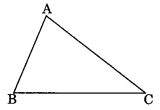3. Scalene triangle: If all sides of the triangle are unequal, then it is called scalene triangle.
AB ≠ BC ≠ CA4. Isosceles triangle: A triangle in which any two sides are equal is called isosceles triangle. Angle opposite to equal sides are also equal to each other.
In ΔABC, AB = AC and ∠ABC = ∠ACB5. Equilateral triangle: A triangle in which all sides are of equal length is called equilateral triangle. Each angle is equal to 60°. In ΔABC, AB = BC = AC and ∠A = ∠B = ∠C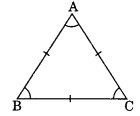6. Acute angled triangle: A triangle having all angles less than 90° is called acute angled triangle.
In ΔABC, ∠A = ∠90°, ∠B = ∠90° and ∠C = ∠90°.7. Obtuse angled triangle: A triangle having one of its three angles is more than 90° is called obtuse angled triangle.
In ΔABC, ∠ABC > 90°8. Right angled triangle: A triangle having its one angle equal to 90° is called right angled triangle.
In ΔABC, ∠B – 90°9. Pythagoras properties: In a right angled triangle, the square of the hypotenuse is equal to the sum of the square of the other sides. In ΔPQR, ∠Q = 90° and PR2 = PQ2 + QR2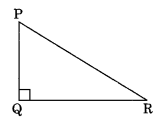10. Median of a triangle: Line segment joining a vertex to the mid-point of its opposite side in a triangle is called the median of the triangle.
In ΔABC, D is the mid-point of BC and AD is the median.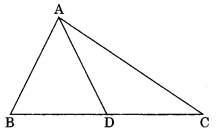11. Altitude of a triangle: Perpendicular drawn from any vertex to the opposite side of a triangle is called its altitude.